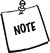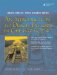# Resource Sharing

Garbage collection is a process that recovers heap memory that is no longer being referenced. Languages such as LISP, Smalltalk, and Java have built-in garbage collectors that run in the background and track object references. When an object is no longer referenced it is deleted, and the memory that it occupied is made available for use by other objects.

The next examples show a way of building garbage collection into the design of a class by means of reference counting. Reference counting is an example of resource sharing.

Each object keeps track of its active references. When an object is created, its reference counter is set to 1. Each time the object is newly referenced, the reference counter is incremented. Each time it loses a reference, the reference counter is decremented. When the reference count becomes 0, the shared object can be deallocated.If the object is about to be changed (e.g., a non-const member function is called) and its reference count is greater than 1, it must be cloned first so that it is no longer shared.

In Example 24.2, we define a homemade string class, MyString, that contains a private inner class, MyStringPrivate, which is responsible for the creation of dynamic arrays and for maintaining a reference count.

An inner class is simply a class defined inside another class. Inner classes are "private" classes, meant to be used only by the containing class.

##### Example 24.2. src/mystring/refcount/refcount.h

 ```[ . . . . ] class MyString { class MyStringPrivate { friend class MyString; <-- 1 public: MyStringPrivate() : m_Len(0), m_RefCount(1) { m_Chars = new (nothrow) char ; m_Chars = 0; } MyStringPrivate(const char* p) : m_RefCount(1) { m_Len = strlen(p); m_Chars = new (nothrow) char[m_Len + 1]; if (m_Chars) strncpy(m_Chars, p, m_Len + 1); else cerr << "Out of memory in MyStringPrivate ctor!" << endl; } ~MyStringPrivate() { delete []m_Chars; } private: int m_Len, m_RefCount; char* m_Chars; }; public: MyString() : m_Impl(new MyStringPrivate) {} MyString(const char* p) : m_Impl(new MyStringPrivate(p)) {} MyString(const MyString& str); ~MyString(); void operator=(const MyString& str); void display() const ; int length() const; private: MyStringPrivate* m_Impl; }; [ . . . . ] ``` (1)Even though this is an inner class, we need to give friend permissions to the containing class.

## nothrow

We used the nothrow qualifier for new (Section 22.9.3) to avoid having to add exception handling code to the example.

The public class MyString, because it manages shared instances of MyStringPrivate, is sometimes called a handler class. It is responsible for maintaining the correct value of the reference counter, and for deleting the pointer when the counter reaches zero.

In Example 24.3, we have output statements in the definitions of three member functions to show the reference counter as objects are created and destroyed.

##### Example 24.3. src/mystring/refcount/refcount.cpp

 ```[ . . . . ] MyString::MyString(const MyString& str) : m_St(str.m_St) { m_St -> m_RefCount++; cout << m_St->m_S << "::refcount: " << m_St->m_RefCount << endl; } MyString::~MyString() { cout << m_St->m_S << "::refcount: " << m_St->m_RefCount << endl; if (--m_St -> m_RefCount == 0) { cout << m_St->m_S << "::memory released" << endl; delete m_St; } } void MyString::operator=(const MyString& str) { if (str.m_St != m_St) { if (--m_St -> m_RefCount == 0) delete m_St; m_St = str.m_St; <-- 1 ++(m_St->m_RefCount); } } [ . . . . ] ``` (1)Just copy the address.

The client code shown in Example 24.4 contains a function with a value parameter and a main() with an inner block. Inside the block, objects are created, copied, and destroyed.

##### Example 24.4. src/mystring/refcount/refcount-test.cpp

 ```#include "refcount.h" void fiddle(MyString lstr1) { cout << "inside fiddle()" << endl; MyString lstr2(lstr1); MyString lstr3; lstr3 = lstr2; } int main() { MyString str1("AABBCCDD"); { cout << "local block begins" << endl; MyString str2(str1); fiddle(str2); cout << "back from fiddle()" << endl; } cout << "local block ends" << endl; str1.display(); cout << endl; return 0; } ```

The output that follows dispassionately shows the entire saga of birth and death as the process races from opening brace to closing brace.

```local block begins
AABBCCDD::refcount: 2
AABBCCDD::refcount: 3
inside fiddle()
AABBCCDD::refcount: 4
AABBCCDD::refcount: 5
AABBCCDD::refcount: 4
AABBCCDD::refcount: 3
back from fiddle()
AABBCCDD::refcount: 2
local block ends
AABBCCDD
AABBCCDD::refcount: 1
AABBCCDD::memory released
src/mystring>
```

#### Exercises: Resource Sharing

 1 Example 24.3 demonstrates reference counting but does not deal with the question of what to do if a MyString object needs to have its value changed when its reference counter is greater than 1. How would you implement the cloning of a MyString object when necessary (but only when necessary)? Implement your solution and test it. 2 Implement a thread-safe version using QMutex. 3 Rewrite MyString using QSharedData and QSharedDataPointer.An Introduction to Design Patterns in C++ with Qt 4
ISBN: 0131879057
EAN: 2147483647
Year: 2004
Pages: 268